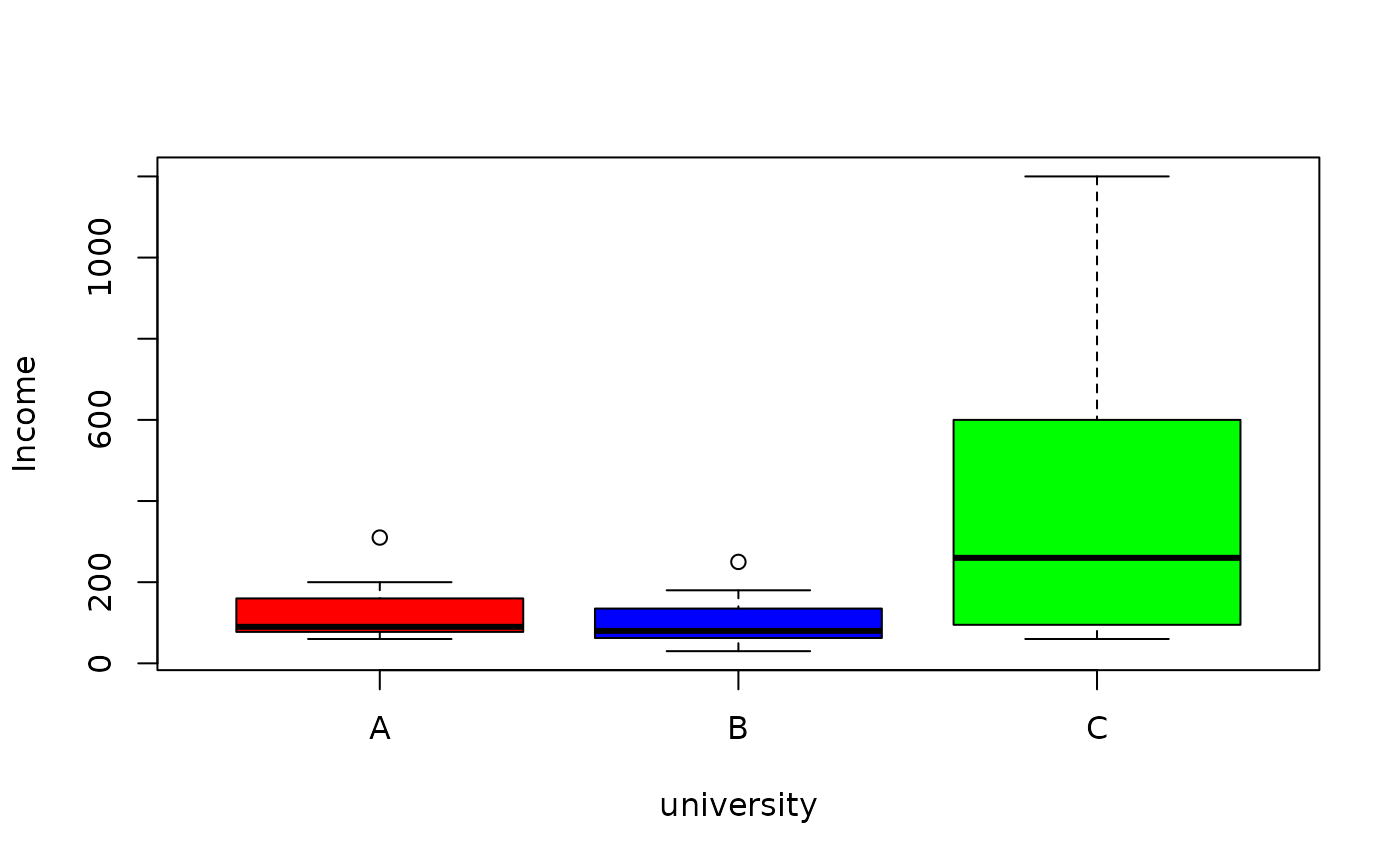Data for Exercise 10.14

Board

## Format

A data frame/tibble with 7 observations on three variables

salary

1999 salary (in $1000) for board directors university a factor with levels A, B, and C ## Examples  boxplot(salary ~ university, data = Board, col = c("red", "blue", "green"), ylab = "Income")tapply(Board$salary, Board$university, summary) #>$A
#>    Min. 1st Qu.  Median    Mean 3rd Qu.    Max.
#>    60.0    77.5    90.0   133.6   160.0   310.0
#>
#> $B #> Min. 1st Qu. Median Mean 3rd Qu. Max. #> 30.0 62.5 80.0 107.9 135.0 250.0 #> #>$C
#>    Min. 1st Qu.  Median    Mean 3rd Qu.    Max.
#>    60.0    95.0   260.0   415.7   600.0  1200.0
#>
anova(lm(salary ~ university, data = Board))
#> Analysis of Variance Table
#>
#> Response: salary
#>            Df  Sum Sq Mean Sq F value  Pr(>F)
#> university  2  408431  204215   2.815 0.08635 .
#> Residuals  18 1305800   72544
#> ---
#> Signif. codes:  0 ‘***’ 0.001 ‘**’ 0.01 ‘*’ 0.05 ‘.’ 0.1 ‘ ’ 1
if (FALSE) {
library(dplyr)
dplyr::group_by(Board, university) %>%
summarize(Average = mean(salary))
}